Can you please explain number 5 and 8 on the working with advanced system of equations.

Sure! (And sorry! I see you submitted this a few days ago but for some reason I’m only seeing it now.)

Since #5 is a no-calc question, let’s get right to solving for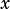by substituting.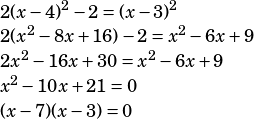So we know the equations intersect atand. Let’s plug those-values into one of the equations to get-values.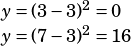The two points we need, then, are (3, 0) and (7, 16). Get the slope:For #8, you can use your calculator to play around here, plugging in values for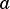and, but the concept being tested is that you have two parabolas with vertices at (5, 5) and (5, 6), and the coefficients(for the upper) and(for the lower) tell you how wide those parabolas will be. Remember, the greater a positive coefficient, the thinner the parabola will be.

If the lower parabola is skinnier than the upper one (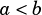), they’ll definitely intersect. However, if they’re both exactly as wide (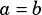), they’ll never intersect, and if the lower one is wider than the upper one (), they’ll never intersect.